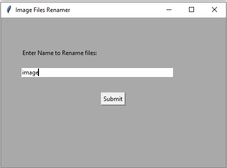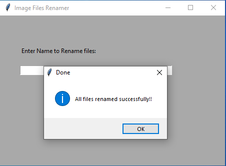# Rename a folder of images using Tkinter

Prerequisites: Python GUI – tkinter, os.listdir() method, os.rename() method

In this article, the task is to rename a batch of images using Python. Now say given n images in a folder having random names. For example, consider the images below:Now the requirement is to rename them in ordered fashion and appending their original dimensions of respective image like `image-0-362x326, image-1-351x414`, …and so on. Doing this manually would be a tedious task but this target can be achieved using the `rename()` and `listdir()` methods in os module.

### rename() method

In Python 3, `rename()` method is used to rename a file or directory. This method is a part of the os module and comes extremely handy.

Syntax: os.rename(src, dst)

Parameters:
src: It is the source address of the file to be renamed
dst: It is thw destination with the new name.

### listdir() method

The listdir method lists out all the content of a given directory.

Syntax: list = os.listdir(‘Src’)

Parameters:
Src: It is the source to be listed out

The following code will do the job for us. Using the Tkinter module it will ask for the folder path in which all the images are stored. It traverses through the lists of all the images in xyz folder, defines the destination (dst), and source (src) addresses and renames using rename() method.

Below is the implementation.

 `# Python 3 code to rename multiple image ` `# files in a directory or folder  ` ` `  ` `  `import` `os   ` `from` `tkinter ``import` `messagebox ` `import` `cv2 ` `from` `tkinter ``import` `filedialog ` `from` `tkinter ``import` `*`  ` `  ` `  `height1 ``=` `0` `width1 ``=` `0` ` `  `# Function to select folder to rename images ` `def` `get_folder_path(): ` `     `  `    ``root ``=` `Tk() ` `    ``root.withdraw() ` `    ``folder_selected ``=` `filedialog.askdirectory() ` `     `  `    ``return` `folder_selected ` ` `  ` `  `# Function to rename multiple files ` `def` `submit(): ` `     `  `    ``source ``=` `src_dir.get() ` `    ``src_dir.``set``("") ` `    ``global` `width1 ` `    ``global` `height1 ` `     `  `    ``input_folder ``=` `get_folder_path() ` `    ``i ``=` `0` `     `  `    ``for` `img_file ``in` `os.listdir(input_folder): ` ` `  `        ``file_name ``=` `os.path.splitext(img_file)[``0``] ` `        ``extension ``=` `os.path.splitext(img_file)[``1``] ` ` `  `        ``if` `extension ``=``=` `'.jpg'``: ` `            ``src ``=` `os.path.join(input_folder, img_file) ` `            ``img ``=` `cv2.imread(src) ` `            ``h, w, c ``=` `img.shape ` `            ``dst ``=` `source ``+` `'-'` `+` `str``(i) ``+` `'-'` `+` `str``(w) ``+` `"x"` `+` `str``(h) ``+` `".jpg"` `            ``dst ``=` `os.path.join(input_folder, dst) ` ` `  `            ``# rename() function will rename all the files ` `            ``os.rename(src, dst) ` `            ``i ``+``=` `1` `             `  `    ``messagebox.showinfo(``"Done"``, ``"All files renamed successfully !!"``) ` ` `  ` `  ` `  `# Driver Code ` `if` `__name__ ``=``=` `'__main__'``: ` `    ``top ``=` `Tk() ` `    ``top.geometry(``"450x300"``) ` `    ``top.title(``"Image Files Renamer"``) ` `    ``top.configure(background ``=``"Dark grey"``) ` ` `  `    ``# For Input Label ` `    ``input_path ``=` `Label(top,  ` `                       ``text ``=``"Enter Name to Rename files:"``, ` `                       ``bg ``=``"Dark grey"``).place(x ``=` `40``, y ``=` `60``) ` `     `  `    ``# For Input Textbox ` `    ``src_dir ``=` `StringVar() ` `    ``input_path_entry_area ``=` `Entry(top, ` `                                  ``textvariable ``=` `src_dir, ` `                                  ``width ``=` `50``).place(x ``=` `40``, y ``=` `100``) ` ` `  `     ``# For submit button ` `    ``submit_button ``=` `Button(top,  ` `                           ``text ``=``"Submit"``, ` `                           ``command ``=` `submit).place(x ``=` `200``, y ``=` `150``) ` ` `  `    ``top.mainloop() `

Output :Attention geek! Strengthen your foundations with the Python Programming Foundation Course and learn the basics.

To begin with, your interview preparations Enhance your Data Structures concepts with the Python DS Course.

My Personal Notes arrow_drop_upCheck out this Author's contributed articles.

If you like GeeksforGeeks and would like to contribute, you can also write an article using contribute.geeksforgeeks.org or mail your article to contribute@geeksforgeeks.org. See your article appearing on the GeeksforGeeks main page and help other Geeks.

Please Improve this article if you find anything incorrect by clicking on the "Improve Article" button below.

Article Tags :

1

Please write to us at contribute@geeksforgeeks.org to report any issue with the above content.